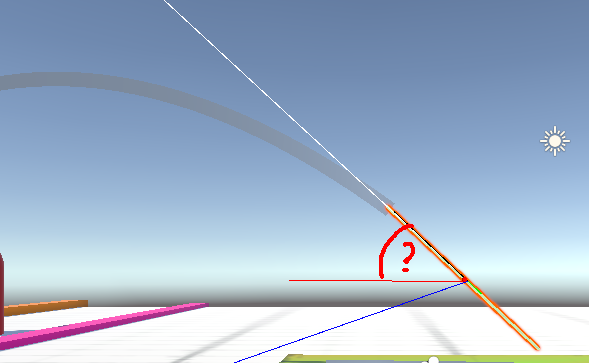# Vector3.Angle is wrong

I am trying to get the throwing angle of an object thrown in VR. The white line is the velocity of the controller, I am taking an average of the last frames here to describe the direction the controller is moving.
While throwing, I want to get the angle between the avg. velocity and the horizontal forward direction (red line). However, my code reports the wrong angles, no matter what I try.

In the example picture, the reported angle is 11 deg. My code is:

``````Vector3 angleOne = AttachedHand.velocity.normalized;
Vector3 angleTwo = new Vector3(AttachedHand.velocity.x, this.transform.position.y, AttachedHand.velocity.z);

//float angle = Vector3.Angle(angleOne, angleTwo);

float angle = Mathf.Atan2(Vector3.Magnitude(Vector3.Cross(angleOne, angleTwo)), Vector3.Dot(angleOne, angleTwo));``````new Vector3(AttachedHand.velocity.x, 0, AttachedHand.velocity.z);